Inclined Plane

# Objective:

1. To find the downward force along an inclined plane, acting on a roller due to the gravitational pull of the earth.
2. To study its relationship with the angle of inclination θ by plotting a graph between applied force and sin θ.

# Theory:

The inclined plane consists of a smooth plane hinged to a base so that it can be set at any desired angle. Consider a heavy metal roller connected to a scale pan by a light extensible string passing over a frictionless pulley resting on the plane as shown in the figure given below.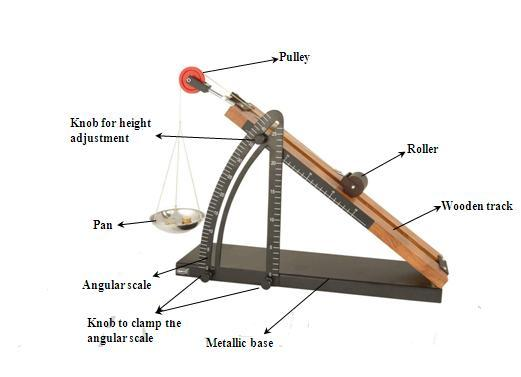If a body of mass (say m) is placed over an inclined plane, that is inclined at an anglewith the horizontal, its weight mg acts vertically downward. The component mg cosof the weight acts normally downward on the plane balances the upward normal reaction (say R) of the inclined plane. The component mg sinof the weight acting parallel to the inclined plane downwards, produces motion in the body.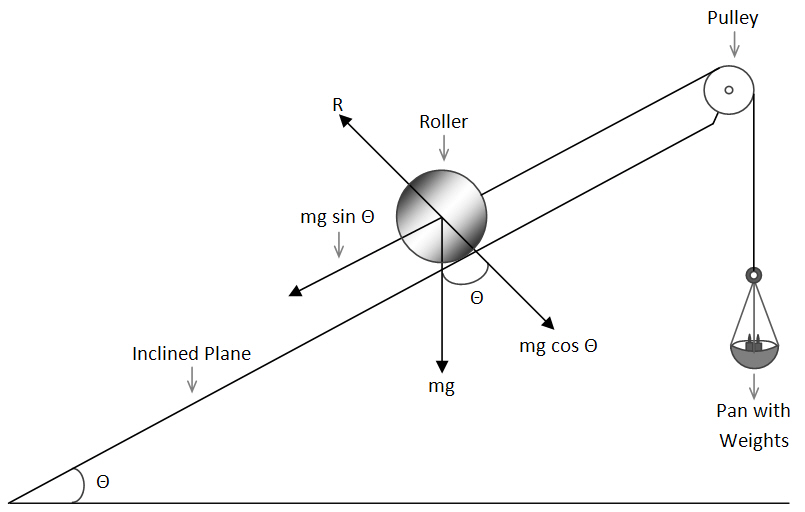If total weight W1=m1g moves the body up and total weight W2 = m2g makes the body move down,

Then the downward force acting on the body along the inclined plane,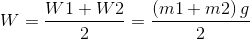which must be equal to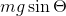ie;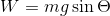--------------(1)

For a constant mass,m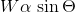Thus, a graph between sin θ along X-axis and W along Y-axis must be a straight line.

# Learning Outcomes:

•     Students understand the working of an inclined plane.
•     Students understand idea of normal reaction and downward force acting on an inclined plane.